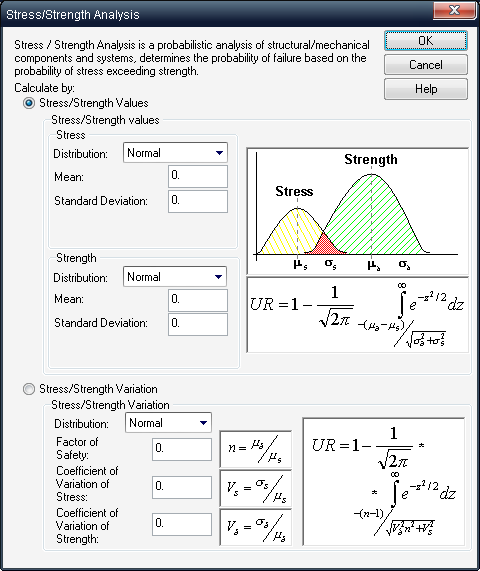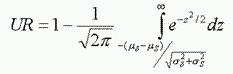### Stress/Strength

Stress/Strength Analysis is a Structural/Mechanical Analysis of Components and Systems.
Stress/Strength analysis method determines the probability of a failure based on the probability of stress exceeding strength.

Calculation of Failure Probability (Unreliability) is performed by selecting one of the two options:

• Distributions of Stress and Strength
• Variation information between Stress and Strength (Factor of Safety n and Variations)Having distributions of Stress and Strength, we may calculate the failure probability (Unreliability) using the following expression:Having variation information between stress and strength (Factor of Safety n and Variations), we may also calculate the Unreliability using another expression: